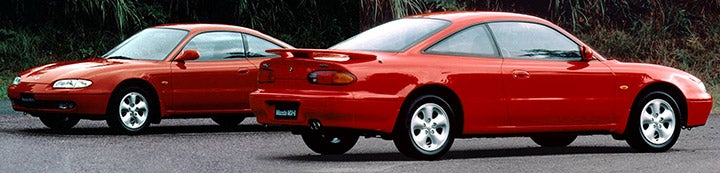1 - 1 of 1 Posts

#### STW

·
Joined
·
2,003 Posts
Discussion Starter · ·
Ever wondered how much there is power going through the little shaft within the turbo? (I did, actually, damn trivia kept me awake the whole night) It's actually easy to calculate.

Let's first pick a familiar IHI RHF5 turbo, here's a map:
http://www.rbracing-rsr.com/turbo/TurboMaps/ihi_maps.gif

It says about 12m^3/min at 2.3 pressure ratio. Working it out in SI units we get 0.2m^3/s and 130kPa above ambient pressure. Pascal is an unit of pressure, defined as newtons per square meter. Thus we can express pressure as 130 000 N/m^2.

Calculating the factors together

0.2m^3/s * 130 000 N/m^2 equals to

26 000 Nm/s

Now since Newton meter, besides being the metric unit of torque, is also the definition joule, unit of energy. Thus we get 26 000 joules per second, or 26kW. Converted to more traditional figures it's 35hp. Efficiency at the said point is likely around 65%, so the actual shaft HP going on in turbo is 35 hp / 0.65 = 54 hp.

Not too bad out of waste energy!

How about a BIG turbo then? Here's a map for a Garrett GT60
http://www.rbracing-rsr.com/turbo/TurboMaps/GT60compress.jpg

150 lbs/min, P/R 3.0, eff. 76%. First the conversion to SI units. At std air density 1.22kg/m^3 and 1lb=0.454kg the flow works out as ~56 cubic meters per minute, or 0.933 m^3/s.

Boost is 2 bars, or 200 kPa.

Second verse, same as the first.
200 000 N/m^2 * 0.933 m^3/s = 186 600 Nm/s

At 76% efficiency, the needed shaft power is 187/0.76 = 246kW, or ~330hp

1 - 1 of 1 Posts